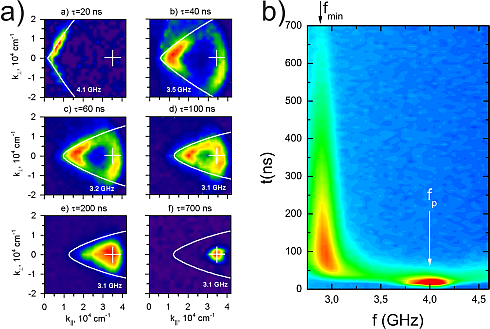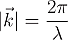# c. What are the signatures of a magnon Bose Einstein Condensation?

The value of the chemical potential after thermalization is determined by the power of the microwave field. So at a critical microwave power the chemical potential reaches the value of the lowest magnon energy in the system. At this point Bose-Einstein condensation can be observed.In the above figure we see experimental data taken at one spatial point of a thin YIG film. Magnons have two important properties. The wave vector k, which is associated with their wavelength and propagation direction and the frequency with which the individual spins are precessing. The frequency coincides with the energy of the corresponding magnon as pointed out in a previous section.

The energy of additionally excited magnons is determined only through the frequency of the microwave field.  In experiments normally single frequency microwave sources are used, so that all injected magnons have one energy.

In Figure b) the energy evolution of magnons at one spatial point of a thin YIG film is presented. At t=0ns a microwave field with a duration of 10 nanoseconds is applied to the YIG-film. A few nanoseconds later we see a strong increase of magnon number at f=4GHz. These are the additionally created magnons which have very narrow energy distribution. It can also be seen, that within a short time period, about 70ns, the additionally created magnons distribute over all observed energies due to scattering between magnons. During this thermalization process most magnons accumulate at the lowest energy state at f=2.9GHz. This relies on the fact that, in this experiment, a sufficient number of additional magnons were introduced so that Bose Einstein condensation could take place after the energy distribution has become thermal again. We can note that one important signature for mBEC is the collection of magnons in the lowest energy state in analogy to atom BEC. They stay there, before they get dissipated into heat after a certain time.

In Figure a) the evolution of the wave vector k of magnons at one spatial point can be seen. The wave vector contains two important pieces of information, the first one being the propagation direction of magnons with respect to an external magnetic field. In the plot k|| denotes propagation parallel to the external magnetic field and k^ perpendicular to it. All angles in between are also allowed. The absolute value of k is simply defined asWe see in this figure, that right after the microwave pulse, induced magnons have a rather narrow wavevector distribution. (The time values on top of the plots are with respect to the onset of a microwave field with 10ns duration.) Forty nanoseconds later the velocity distribution is broadened due to scattering, the magnon gas begins to “thermalize” e.g. becoming a thermal wavevector distribution. As in this experiment the induced number of magnons was sufficient to achieve BEC, the distribution begins to become narrower during the thermalization process. This is another strong indication for mBEC. In the end most magnons collect at one point in k-space.

Another interesting question, that concerns properties of a Magnon Bose Einstein kondensate, is:

How does coherence manifest in Magnon Bose Einstein Condensates?